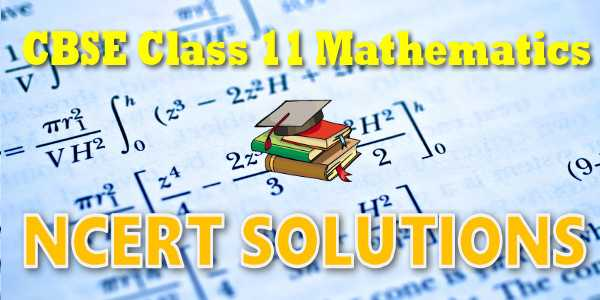No products in the cart.

# NCERT solutions for class 11 Mathematics## CBSE, JEE, NEET, CUET

Question Bank, Mock Tests, Exam Papers

NCERT Solutions, Sample Papers, Notes, Videos

## Class 11 Maths NCERT solutions PDF

As you know CBSE recommends NCERT Books for class 12. That's why how textbook solutions is very important for studies and for your exam. CBSE board exam are based on NCERT curriculum most of the question are asked from these books. So, don't worry we are here you to provide NCERT solutions for class 11 Mathematics book solutions are available in PDF format for free download. These Chapter wise NCERT solutions for class 11 Mathematics solved by expert and experienced teachers at myCBSEguide. You can download Maths NCERT Solutions for all the chapters in our mycbseguide website and mobile app for free.
Sets
Exercise 1.1
Exercise 1.2
Exercise 1.3
Exercise 1.4
Exercise 1.5
Exercise 1.6
Miscellaneous## myCBSEguide App

Complete Guide for CBSE Students

NCERT Solutions, NCERT Exemplars, Revison Notes, Free Videos, CBSE Papers, MCQ Tests & more.

Relations and Functions
Exercise 2.1
Exercise 2.2
Exercise 2.3
Miscellaneous
Trigonometric Functions
Exercise 3.1
Miscellaneous
Exercise 3.2
Exercise 3.3
Exercise 3.4
Exercise 5.1
Exercise 5.2
Exercise 5.3
Miscellaneous
Linear Inequalities
Exercise 6.1
Exercise 6.2
Exercise 6.3
Miscellaneous
Permutations and Combinations
Exercise 7.1
Exercise 7.2
Exercise 7.3
Exercise 7.4
Miscellaneous
Binomial Theorem
Exercise 8.1
Exercise 8.2
Miscellaneous
Sequences and Series
Exercise 9.1
Exercise 9.2
Exercise 9.3
Exercise 9.4
Miscellaneous
Straight Lines
Exercise 10.1
Exercise 10.2
Exercise 10.3
Miscellaneous
Conic Sections
Exercise 11.1
Exercise 11.2
Exercise 11.3
Exercise 11.4
Miscellaneous
Introduction to 3D Geometry
Exercise 12.1
Exercise 12.2
Exercise 12.3
Miscellaneous
Limits and Derivatives
Exercise 13.1
Miscellaneous
Exercise 13.2
Statistics
Exercise 15.1
Exercise 15.2
Exercise 15.3
Miscellaneous
Probability
Exercise 16.1
Exercise 16.2
Exercise 16.3
Miscellaneous### Student Subscription

##### Unlock the exclusive content designed for the toppers## myCBSEguide App

Complete Guide for CBSE Students

NCERT Solutions, NCERT Exemplars, Revison Notes, Free Videos, CBSE Papers, MCQ Tests & more.## NCERT Class 11 Maths Textbook Solutions

Maths ncert solutions contains 16 chapters. If you are preparing for entrance exam like JEE Mains you need to do lots of practice of Mathematics and have a deep knowledge of this subject .The class 11 maths NCERT solutions helpful for your exam to score well.

## NCERT Class 11 Maths Chapters

Chapter 1 - Sets
Chapter 2 - Relations and Functions
Chapter 3 - Trigonometric Functions
Chapter 4 - Principle of Mathematical Induction
Chapter 5 - Complex Numbers and Quadratic Equations
Chapter 6 - Linear Inequalities
Chapter 7 - Permutations and Combinations
Chapter 8 - Binomial Theorem
Chapter 9 - Sequences and Series
Chapter 10 - Straight Lines
Chapter 11 - Conic Sections
Chapter 12 - Introduction to Three Dimensional Geometry
Chapter 13 - Limits and Derivatives
Chapter 14 - Mathematical Reasoning
Chapter 15 - Statistics
Chapter 16 - Probability

## NCERT Solutions for Class 11## myCBSEguide

Trusted by 1 Crore+ Students## Test Generator

Create papers online. It's FREE.## CUET Mock Tests

75,000+ questions to practice only on myCBSEguide app